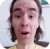FreeKB - Java Programming Form data (POST GET)
Java Programming - Form data (POST GET)GET

Let's say you have a URL with a parameter such as id=12345.

``www.example.com?id=12345``

The following markup uses JSTL, so lets add the following to the head of your JSP page.

``<%@ taglib uri="http://java.sun.com/jsp/jstl/core" prefix="c" %>``

The following will print the id (12345 in this example).

``<c:out value="\${param.id}" />``

Or, you can use c:set to store the value in a variable. In this example, the ID is stored in a variable called "id".

``<c:set var="id" value="\${param.id}" />``

You can then print the value using this markup.

``\${id}``

POST to a JSP page

In this example the value in the form is associated with the "username" value are is POST to example.jsp.

``````<form method="post" action="example.jsp">
</form>``````

The following markup uses JSTL, so lets add the following to the head of your JSP page.

``<%@ taglib uri="http://java.sun.com/jsp/jstl/core" prefix="c" %>``

On example.jsp, use the following to display the username value.

``<c:out value="\${param.username}" />``

Or, you can use c:set to store the value in a variable. In this example, the ID is stored in a variable called "username".

``<c:set var="username" value="\${param.username}" />``

You can then print the value using this markup.

``\${username}``

POST to a Servlet

Let's say you have a servlet that contains the following annotation.

``@WebServlet("/foo")``

In this example the value in the form is associated with the "username" value are is POST to the "foo" servlet.

``````<form method="post" action="foo">
<input type="text" name="bar">
</form>``````

Since the form method is "post", we need to place markup inside of the doPost method in the servlet.

``protected void doPost``

This markup creates a variable called "bar" that will get the value that was put inside of the HTML form.

``String bar = request.getParameter("bar");``

We can then use the "bar" variable.

``System.out.println(bar);	``

We will never share your name or email with anyone. Enter your email if you would like to be notified when we respond to your comment.

Please enter c3ea4 in the box below so that we can be sure you are a human.

Web design by yours truely - me, myself, and I   |   jeremy.canfield@freekb.net   |